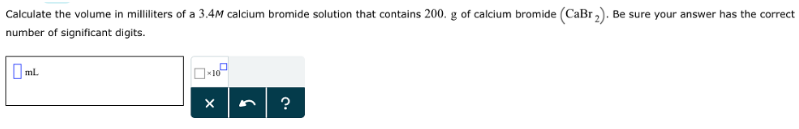# Problem: Calculate the volume in millimeters of a 3.4M calcium bromide solution that contains 200 g of calcium bromide (CaBr2). Be sure your answer has the correct number of significant digits.

###### FREE Expert Solution
84% (327 ratings)###### Problem Details

Calculate the volume in millimeters of a 3.4M calcium bromide solution that contains 200 g of calcium bromide (CaBr2). Be sure your answer has the correct number of significant digits.